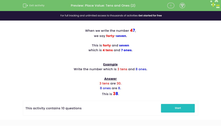# Create Numbers from Tens and Ones

In this worksheet, students will write a number given in tens and ones as a proper number.Key stage:  KS 1

Curriculum topic:   Number: Number and Place Value

Curriculum subtopic:   Recognise Tens and Ones

Difficulty level:#### Worksheet Overview

This activity is about splitting numbers into tens and ones.

When we write the number 47,

we say forty-seven.

This is forty and seven

which is 4 tens and 7 ones.

Example

Write the number which is 3 tens and 8 ones.

3 tens are 30.

8 ones are 8.

This is 38.

Shall we try another one?What number can we make from 5 tens and 2 ones?

5 tens are 50

2 ones are 2.

This makes 52.

Time for some questions now.

### What is EdPlace?

We're your National Curriculum aligned online education content provider helping each child succeed in English, maths and science from year 1 to GCSE. With an EdPlace account you’ll be able to track and measure progress, helping each child achieve their best. We build confidence and attainment by personalising each child’s learning at a level that suits them.

Get started